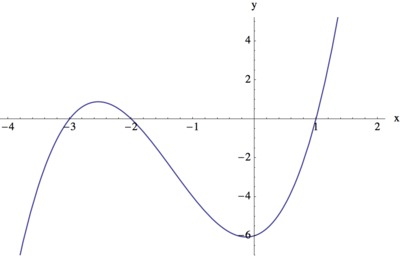# Graphing from Factors III

Alignments to Content Standards: A-APR.B.3 A-APR.B.2

Mike is trying to sketch a graph of the polynomial $$f(x)=x^3+4x^2+x-6.$$ He notices that the coefficients of $f(x)$ add up to zero $(1 + 4 + 1 -6 = 0)$ and says

This means that 1 is a root of $f(x)$, and I can use this to help factor $f(x)$ and produce the graph.
1. Is Mike right that 1 is a root of $f(x)$? Explain his reasoning.
2. Find all roots of $f(x)$.
3. Find all inputs $x$ for which $f(x)\lt 0$.
4. Use the information you have gathered to sketch a rough graph of $f$.

## IM Commentary

The task has students use the remainder theorem to deduce a linear factor of a cubic polynomial, and then to completely factor the polynomial. Students will need some procedure (e.g., synthetic or long division, or guess-and-check the coefficients) for determining the quadratic factor. Having the factored form permits students to deduce much about the structure of the graph. In addition to having learned the $x$-intercepts, writing the polynomial as $$x^3+4x^2+x-6=(x-1)(x+2)(x+3)$$ also reveals, for example, where the function is negative: indeed, to give a negative output, exactly one of the three factors (or all three factors) has to be negative, giving $x\lt -3$ or $-2\lt x\ltÂ 1$.

The task can be the basis for a classroom activity and discussion. In this task students engage in SMP 7 - Look for and make use of structure. Students use the structure of the polynomial in factored form to make deductions about the structure of the graph.Â

## Solution

1. Mike is right that 1 is a root of $f(x)$ and we verify this by evaluating $f(1)$, we also see why his reasoning works:

\begin{align} f(1) &= 1^3 + 4 \times 1^2 + 1 \times 1 - 6 \\ &= 1^3 + 4 + 1 - 6 \\ &= 0. \end{align}
The key here is that when we substitute 1 into $f(x)$, all of the powers of $x$ are 1, and so the value of $f(1)$ is simply the sum of the coefficients of $f(x)$. Since the sum of the coefficients of $f(x)$ is 0 this means that $f(1) = 0$ and so 1 is a root of $f(x)$.

Â

2. By the remainder theorem, since $1$ is a root of $f(x)$, the linear term $x-1$ is a factor of $f$: that is, when we divide $f(x)$ by $x-1$ we know that there will be zero remainder. We find (e.g., by long division, or synthetic division, among other choices) $$f(x) = (x-1)(x^2 + 5x + 6).$$ Since $x^2 + 5x + 6$ factors as $(x+2)(x+3)$, we conclude $$f(x) = (x-1)(x+2)(x+3).$$

3. We can use the factored form of $f(x)$ to deduce where $f$ is positive or negative. Since a product of three real numbers is negative when all three are negative, or when exactly one is negative, $f(x)$ gives a negative output when one of two things happens:

• All of $x-1$, $x+2$, and $x+3$ are negative. Since $x+3$ is the largest of the three, this happens when $x+3\lt 0$, which is when $x\lt -3$.
• Exactly one of the three linear terms is negative. Since $x-1\lt x+2\lt x+3$, this could only be true if $x-1\lt 0$ but $x+2\gt 0$, that is, $-2\lt x\lt 1$.
So $f(x)$ is negative if and only if $-2\lt x\ltÂ 1$ or $x\lt -3$.

Â

4. Since $f(x)$ is a cubic polynomial with positive coefficient for $x^3$ this means that it will take large positive values when $x$ is large and positive and large negative values when $x$ is large and negative. This, together with the knowledge of where $f(x)$ takes the value 0, and where $f(x)$ is negative, allows us to draw the following sketch for the graph of $y=f(x)$: